Reconstruction of the 2020 Norton Plantation formation 1.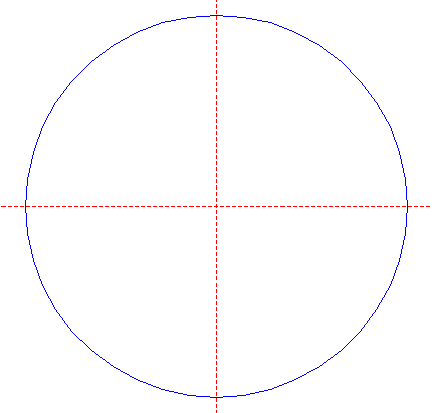Draw a circle. Draw and extend the horizontal and vertical centerlines. 2.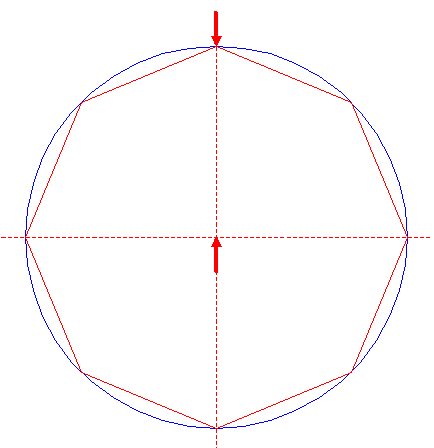Construct the inscribed octagon (regular 8-sided polygon) of circle 1, pointing up. 3.Construct the inscribed circle of octagon 2. 4.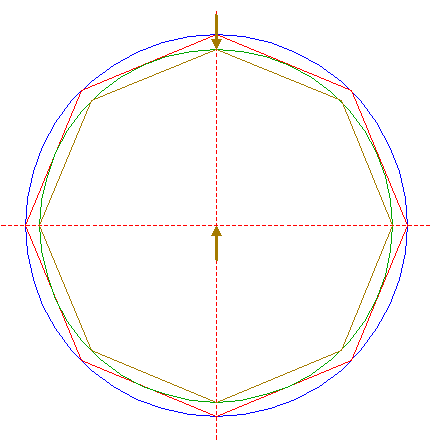Construct the inscribed octagon of circle 3, pointing up. 5.Construct the inscribed circle of octagon 4. 6.Construct the inscribed octagon of circle 5, pointing up. 7.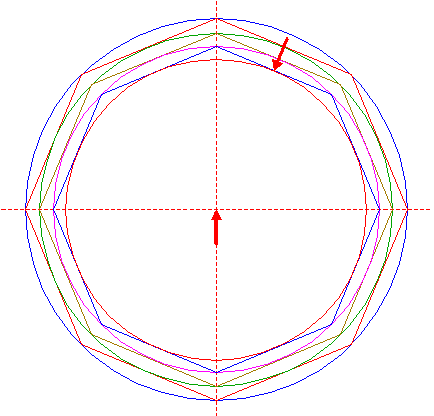Construct the inscribed circle of octagon 6. 8.Construct a circle centered at the top of octagon 6, tangent to the upper sides of octagon 2. 9.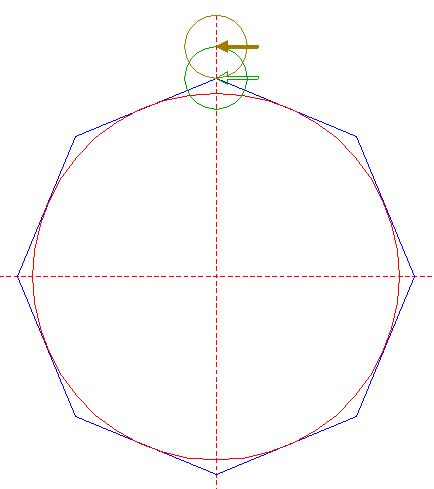Copy circle 8 to its upper intersection with the vertical centerline. 10.Copy circle 9 seven times, to the corresponding positions with respect to the other angular points of octagon 6. 11.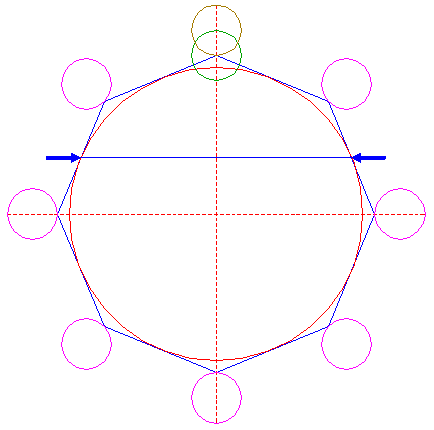Draw the connecting line between the lefthand and righthand intersections (tangent points) of octagon 6 and circle 7, just above the horizontal centerline. 12.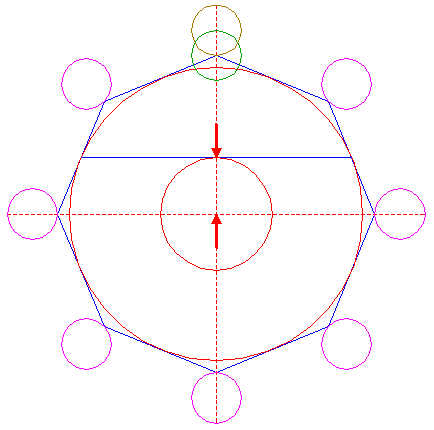Construct a circle concentric to circle 7, tangent to line 11. 13.Construct a circle centered at the lower intersection of circle 8 and the vertical centerline, tangent to circle 12 at the upper side. 14.Copy circle 13 to the center of circle 7. 15.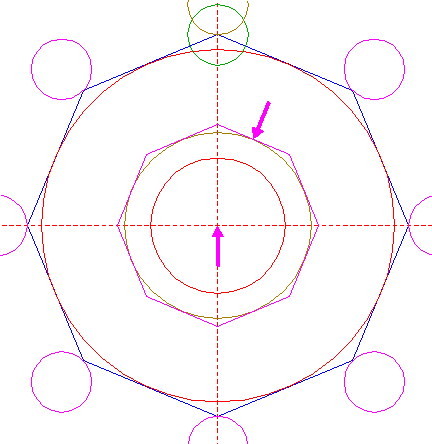Construct the circumscribed octagon of circle 14, pointing up. 16.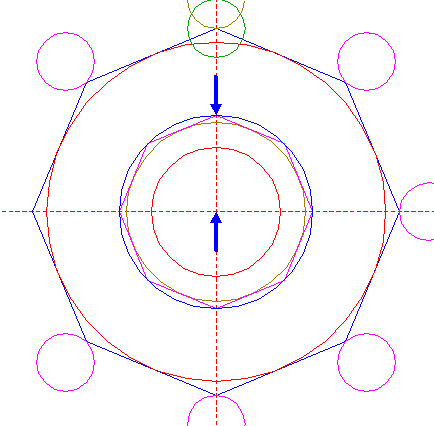Construct the circumscribed circle of octagon 15. 17.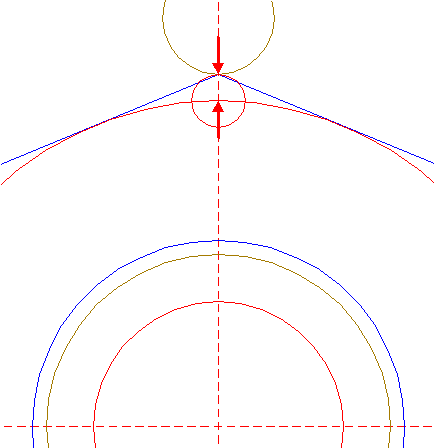Construct a circle centered at the upper intersection of circle 7 and the vertical centerline, tangent to circle 9 at the lower side. 18.Draw the connecting line between two intersections (tangent points) of octagon 6 and circle 7, one at the righthand side just above, the other at the lefthand side just below the horizontal centerline. 19.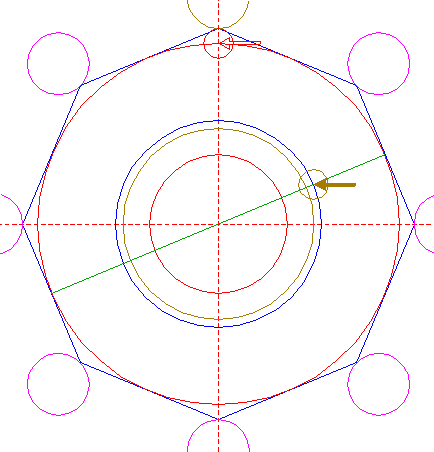Copy circle 17 to the upper righthand intersection of circle 16 and line 18. 20.Construct a circle centered at the upper righthand intersection of circle 19 and line 18, passing through the center of circle 17. 21.Construct a circle centered at the lower lefthand intersection of circle 16 and line 18, tangent to circle 14 at the near side. 22.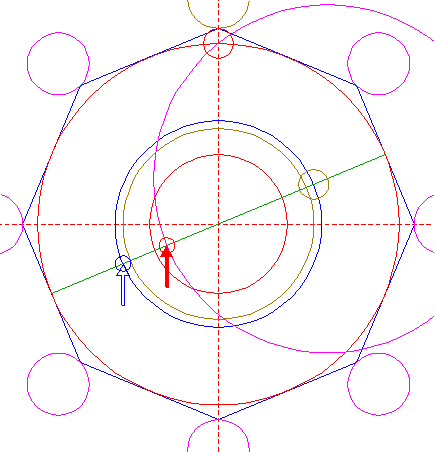Copy circle 21 to the intersection of circle 20 and line 18. 23.Construct a circle concentric to circle 20, tangent to circle 22 at the lower lefthand side. 24.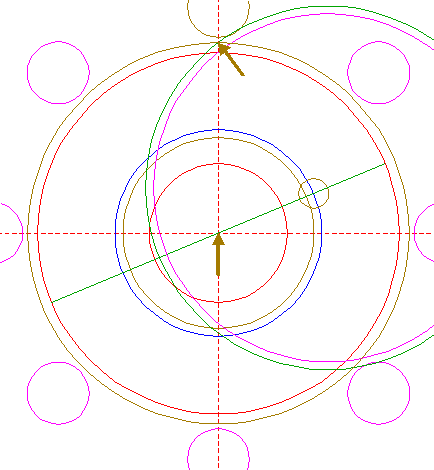Construct a circle concentric to circle 7, passing through the upper intersection of circle 23 and the vertical centerline. 25.Construct a circle concentric to circle 7, tangent to circle 19 at the upper righthand side. 26.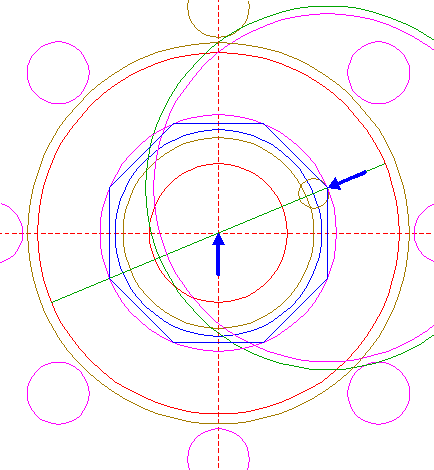Construct the inscribed octagon of circle 25, with an angular point coincident with the center of circle 20. 27.Copy circles 20 and 23 together seven times, to the other angular points of octagon 26. 28.Circles 7, 9, 10, 12, 14, 16, 20, 23, 24, and 27, are used for the final reconstruction. 29.Remove all parts not visible within the formation itself. 30.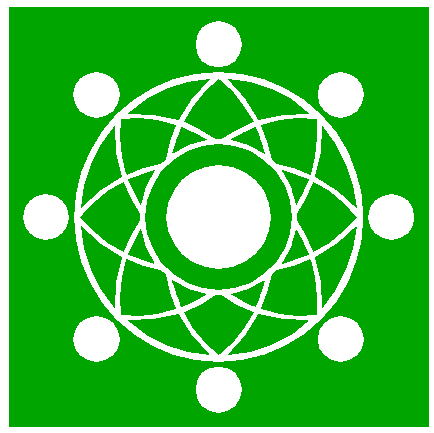Colour all areas corresponding to standing... 31.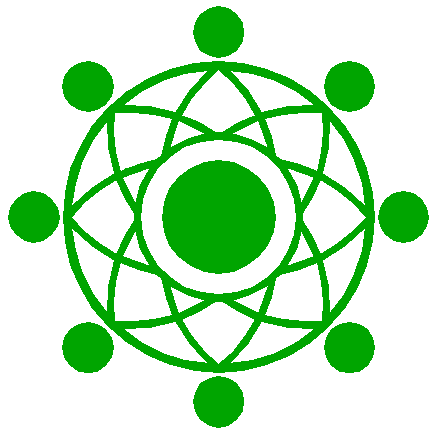...or to flattened crop, and finish the reconstruction of the 2020 Norton Plantation formation. 32.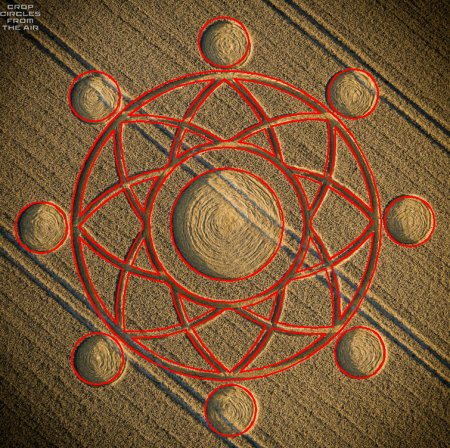The final result, matched with the aerial image.